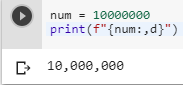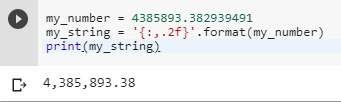2 views
in Python

I am trying to print an integer in Python 2.6.1 with commas as thousands separators. For example, I want to show the number 1234567 as 1,234,567. How would I go about doing this? I have seen many examples on Google, but I am looking for the simplest practical way.

It does not need to be locale-specific to decide between periods and commas. I would prefer something as simple as reasonably possible.

by (106k points)

This can be easily done by using  f-strings:

num = 10000000

print(f"{num:,d}")• In this method, the part after the colon is the format specifier. The comma is the separator character you want,

• This is equivalent of using format(num, ",d") for older versions of python.

• we can use my_string = '{:,.2f}'.format(my_number) to convert float value into commas as thousands separators.

my_number = 4385893.382939491

my_string = '{:,.2f}'.format(my_number)